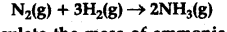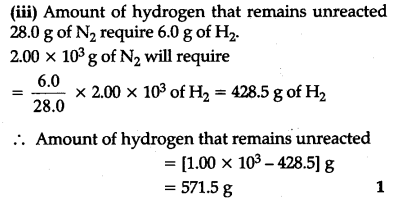# Dinitrogen and dihydrogen react with each other to produce ammonia according to the following chemical equation:

Dinitrogen and dihydrogen react with each other to produce ammonia according to the following chemical equation:(i) Calculate the mass of ammonia produced if
2.00 x \${{10}^{3}}g dinitrogen reacts with 1.00 X {{10}^{3}}\$g of dihydrogen.
(ii) Will any of the reactants remain unreacted ?
(iii) If yes, which one and what would be its mass?